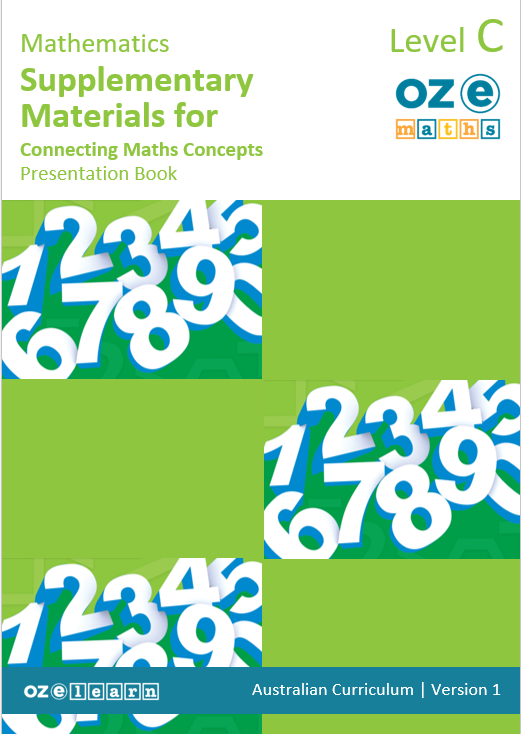## Oz-e-maths Supplementary Materials for Connecting Maths Concepts Years F-5

Top-ups and Swap-outs for Currency and Measurement - Year 2### Overview

Top-ups and Swap-outs for Currency and Measurement – Year 2 is a Mathematics unit for Year 2 students. It works in cooperation with Connecting Maths Concepts (CMC) to ensure that delivery of CMC aligns with Australian Curriculum gap requirements. It aligns to the Australian Curriculum:
• Measure the length of shapes and objects using informal units, recognising that units need to be uniform and used end-to-end (AC9M1M02)
• Describe the duration and sequence of events using years, months, weeks, days and hours (AC9M1M03)
• Group, partition and rearrange collections up to 1000 in hundreds, tens and ones to facilitate more efficient counting (ACMNA028)
• Group, partition, rearrange and rename numbers up to 1000 according to their place value and into other number groupings (AC9M2N02)
• Recognise and explain the connection between addition and subtraction as inverse operations, apply to partition numbers and find unknown (AC9M2A01)
• Count and order small collections of Australian coins and notes according to their value (ACMNA034)
Success Criteria
Top-Ups
• Identify and describe flip and slide
• Identify and describe half and quarter turns to create a pattern
• Use skip counting by 2s, 5s and 10s to count a collection of objects and create patterns
• Understand two-digit and three-digit numbers place value
Swap-Outs
• Identify and count Australian coins
• Apply ruler skills to measure length and count backwards
• To solve comparison word problems
Learning Objectives
In Lesson 3 (CMC Level C: Lesson 31-40) students learn to:
• Know that two-digit numbers are comprised of tens and ones
• Use pictorial MABs to demonstrate that three-digit numbers are comprised of hundreds, tens, and ones/units
• Show that three-digit numbers are comprised of hundreds, tens, and ones/units
• Demonstrate three-digit numbers are comprised of hundreds, tens, and ones/units
In Lesson 4 (CMC Level C: Lesson 51-60) students learn to:
• Describe skip counting and the pattern of counting by 2s
• Use counting by 2s to count a collection of objects
• Represent skip counting by 2s on a number line, identifying the missing number
• Describe the 2s skip counting pattern beyond 100
• Describe the pattern of skip counting by 5s
• Use skip counting by 5s to represent the pattern
• Use skip counting by 10s to represent the pattern and identify the missing number
In lesson 5 (CMC Level C: Lessons 91-100) students learn to:
• Describe the effect of a one-step slide
• Identify a one-step slide
• Describe the effect of a flip
• Identify a flip or slide
• Investigate the effect of flips by moving and drawing shapes
• Demonstrate the effect of slides and flips by drawing irregular shapes
In Lesson 6 (CMC Level C: Lesson 101-110) students learn to:
• Identify and describe half and quarter turns.
• Demonstrate a half turn and quarter turn to create a pattern.
In the Swap Outs students learn to:
• identify and count Australian coins
• apply ruler skills to measure length
• use a ruler to count backwards
• to solve comparison word problems
Assessment
Teaching Resources Top-Ups
• Teaching Guide
• Student Workbook
• Board displays

## Recommended Units

\Learnworlds\Codeneurons\Pages\ZoneRenderers\CourseCards